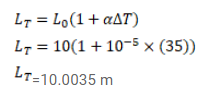# A concrete slab has a length of 10 m on a winter nightQuestion:

A concrete slab has a length of $10 \mathrm{~m}$ on a winter night when the temperature is $0^{\circ} \mathrm{C}$. Find the length of the slab on a summer day when the temperature is $35^{\circ}$. The co-efficient of linear expansion of concrete is $1.0 x^{10^{-5}} /{ }^{\circ} \mathrm{C}$

Solution: